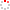Chinese Academy of Sciences Institutional Repositories Grid
###### Measurement of the ratio of branching fractions b(b-c(+) -> j/psi tau(+)nu(tau))/b(b-c(+) -> j/psi mu(+)nu(mu))

 Author Aaij, R.42; Adeva, B.41; Adinolfi, M.50; Ajaltouni, Z.5; Akar, S.61; Albrecht, J.10; Alessio, F.42; Alexander, M.55; Alfonso Albero, A.40,49; Ali, S.45 Source Physical review lettersIssued Date 2018-03-23 Volume 120Issue:12Pages:10 ISSN 0031-9007 DOI 10.1103/physrevlett.120.121801 Corresponding Author Aaij, r.() English Abstract A measurement is reported of the ratio of branching fractions r(j/psi) = b(b-c(+) -> j/psi tau(+)nu(tau))/b(b-c(+) -> j/psi mu(+)nu(mu)), where the tau(+) lepton is identified in the decay mode tthorn tau(+) -> mu(+)nu(mu)(nu) over bar (tau). this analysis uses a sample of proton-proton collision data corresponding to 3.0 fb(-1) of integrated luminosity recorded with the lhcb experiment at center-of-mass energies of 7 and 8 tev. a signal is found for the decay b-c(+) -> j/psi tau(+)nu(tau) at a significance of 3 standard deviations corrected for systematic uncertainty, and the ratio of the branching fractions is measured to be r(j/psi) = 0.71 +/- 0.17(stat) +/- 0.18(syst). this result lies within 2 standard deviations above the range of central values currently predicted by the standard model. WOS Research Area Physics WOS Subject Physics, Multidisciplinary Language 英语 WOS ID WOS:000428167200007 Publisher AMER PHYSICAL SOC URI http://www.irgrid.ac.cn/handle/1471x/2178200 Collection 高能物理研究所 Corresponding Author Aaij, R. Affiliation 1.CBPF, Rio De Janeiro, Brazil2.Univ Fed Rio de Janeiro UFRJ, Rio De Janeiro, Brazil3.Tsinghua Univ, Ctr High Energy Phys, Beijing, Peoples R China4.Univ Savoie Mont Blanc, CNRS, IN2P3, LAPP, Annecy Le Vieux, France5.Clermont Univ, Univ Blaise Pascal, CNRS, IN2P3, Clermont Ferrand, France6.Aix Marseille Univ, CNRS, IN2P3, CPPM, Marseille, France7.Univ Paris Saclay, CNRS, IN2P3, Univ Paris Sud,LAL, Orsay, France8.Univ Paris Diderot, Univ Pierre & Marie Curie, CNRS IN2P3, LPNHE, Paris, France9.Rhein Westfal TH Aachen, Phys Inst 1, Aachen, Germany10.Tech Univ Dortmund, Fak Phys, Dortmund, Germany Recommended CitationGB/T 7714 Aaij, R.,Adeva, B.,Adinolfi, M.,et al. Measurement of the ratio of branching fractions b(b-c(+) -> j/psi tau(+)nu(tau))/b(b-c(+) -> j/psi mu(+)nu(mu))[J]. Physical review letters,2018,120(12):10. APA Aaij, R..,Adeva, B..,Adinolfi, M..,Ajaltouni, Z..,Akar, S..,...&Zucchelli, S..(2018).Measurement of the ratio of branching fractions b(b-c(+) -> j/psi tau(+)nu(tau))/b(b-c(+) -> j/psi mu(+)nu(mu)).Physical review letters,120(12),10. MLA Aaij, R.,et al."Measurement of the ratio of branching fractions b(b-c(+) -> j/psi tau(+)nu(tau))/b(b-c(+) -> j/psi mu(+)nu(mu))".Physical review letters 120.12(2018):10.